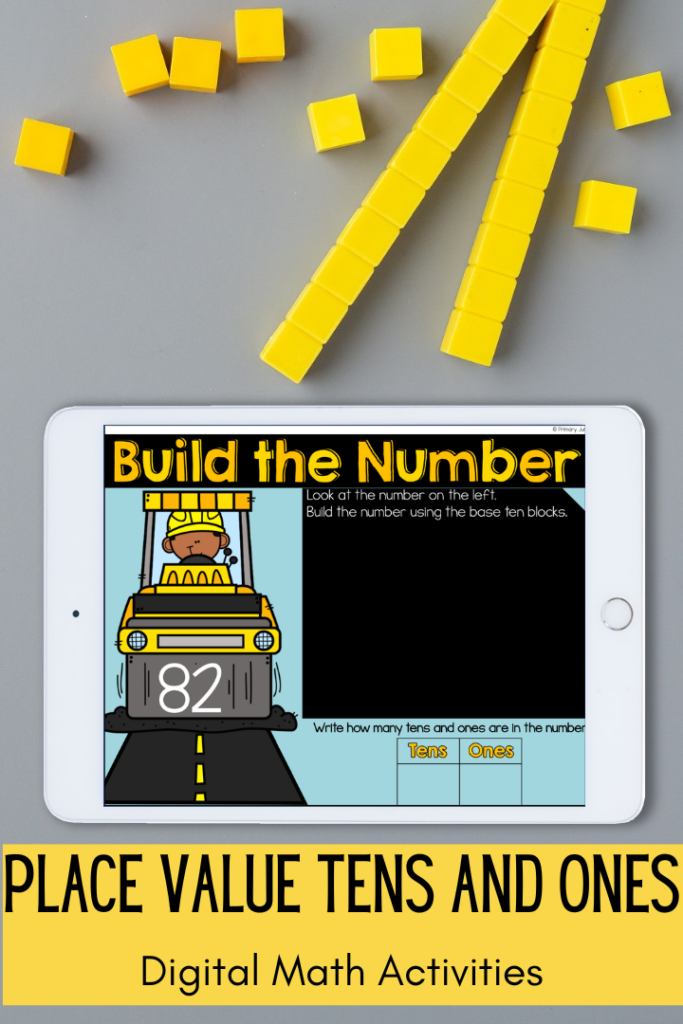# Digital Activities for Place Value Tens and Ones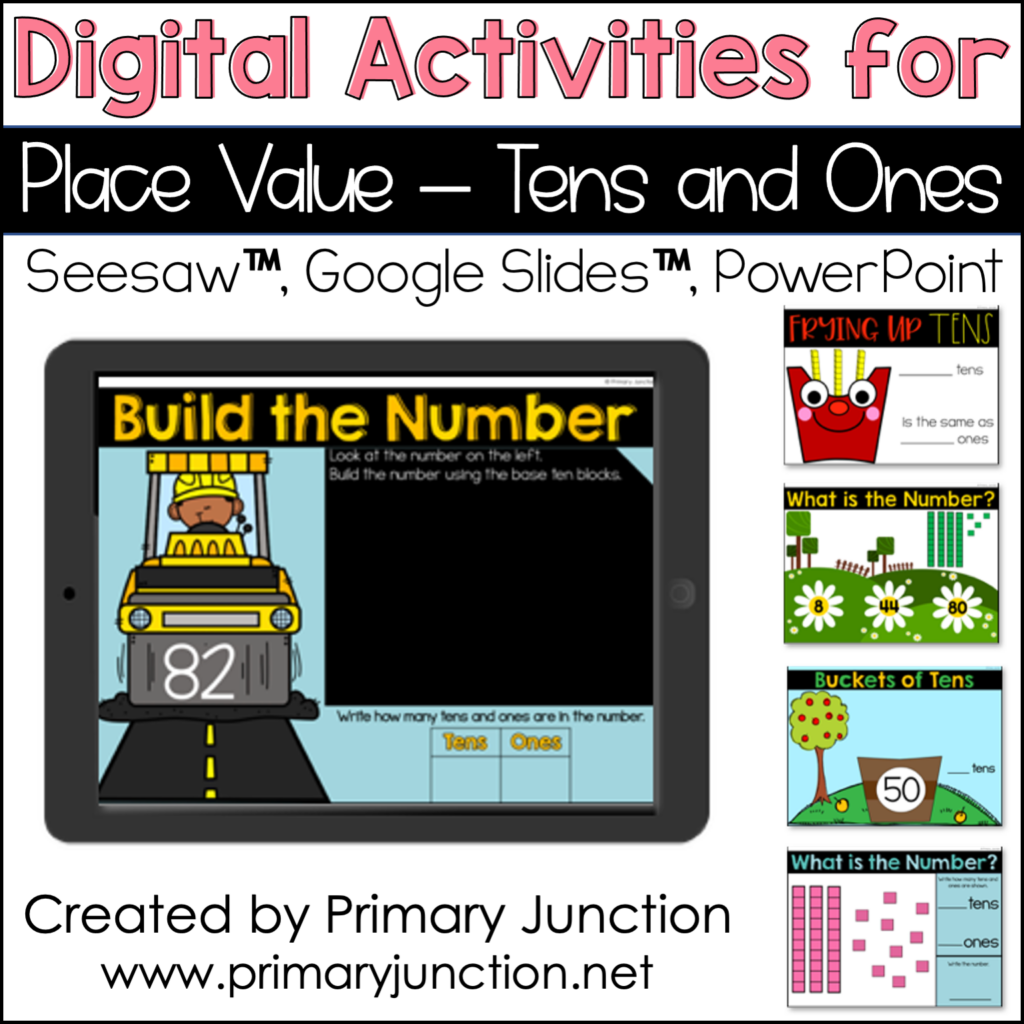Teaching place value and tens and ones skills is one of my favorite math units to teach in First Grade each year!  I believe math skill practice should be fun, so this year, I created some digital activities for my students to practice place value tens and ones skills.  These digital activities are compatible with Seesaw, Google Slides, and Powerpoint.

# Which Place Value Tens and Ones Math Skills are Covered?

This 8 no-prep, digital math activities packet was created with First Grade students in mind.  The included activities are aligned to the First Grade Number and Operations in Base Ten Standards.  With these activities, students have the opportunity to practice the following place value tens and ones skills:  adding tens and ones to compose two-digit numbers, comparing two-digit numbers using tens and ones and greater than, less than, and equal signs, identifying tens in two-digit numbers, building and composing two-digit numbers using base ten blocks, showing and understanding the relationship between tens and ones, and identifying the value of tens and ones.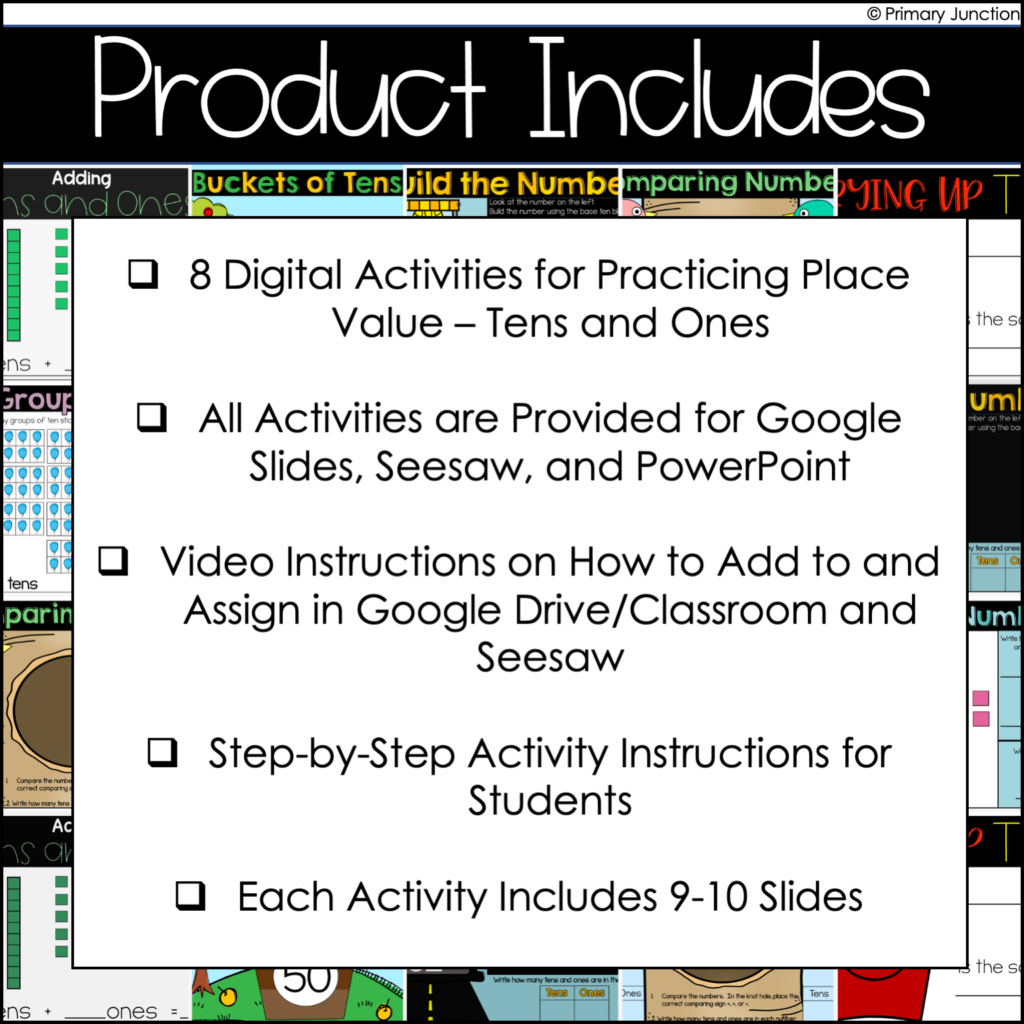## Which Activities Are Included?

1. Adding Tens and Ones – In this activity, students are shown a two-digit number using tens and ones. Students must write how many tens and ones are shown. Then, students must add the tens and ones together and write the number being shown with base ten blocks.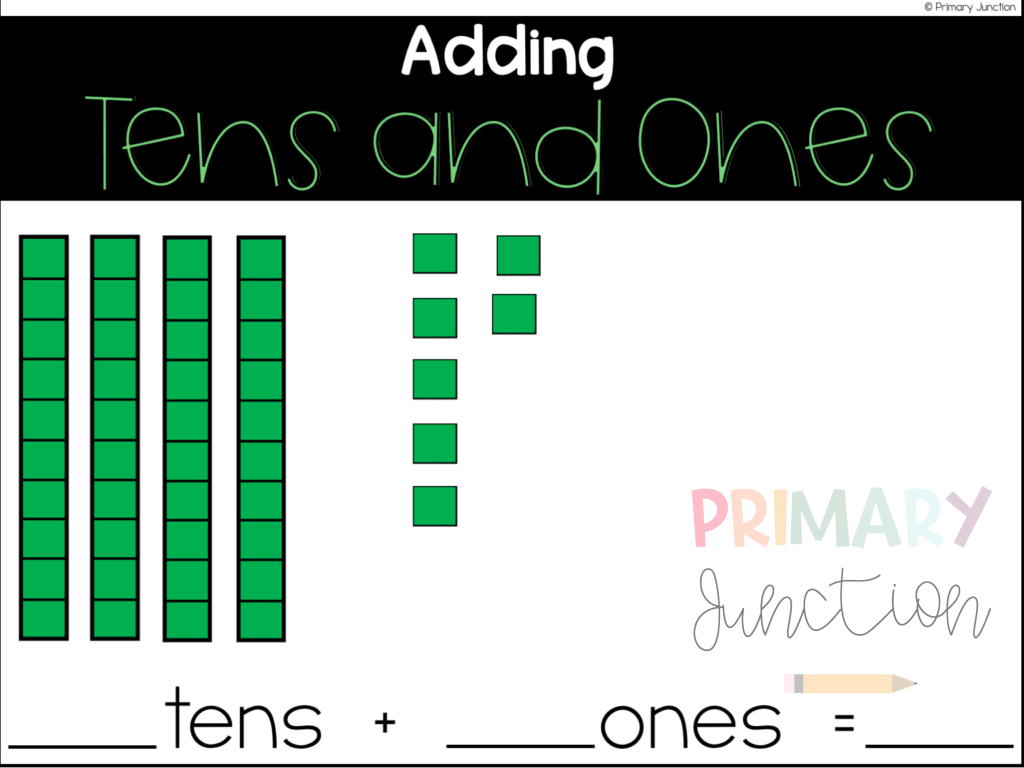2. Comparing Numbers – In this activity, students are shown two, two-digit numbers. Students will need to write the tens and ones in each number and then compare the number using a comparison sign of less than, greater than, or equal.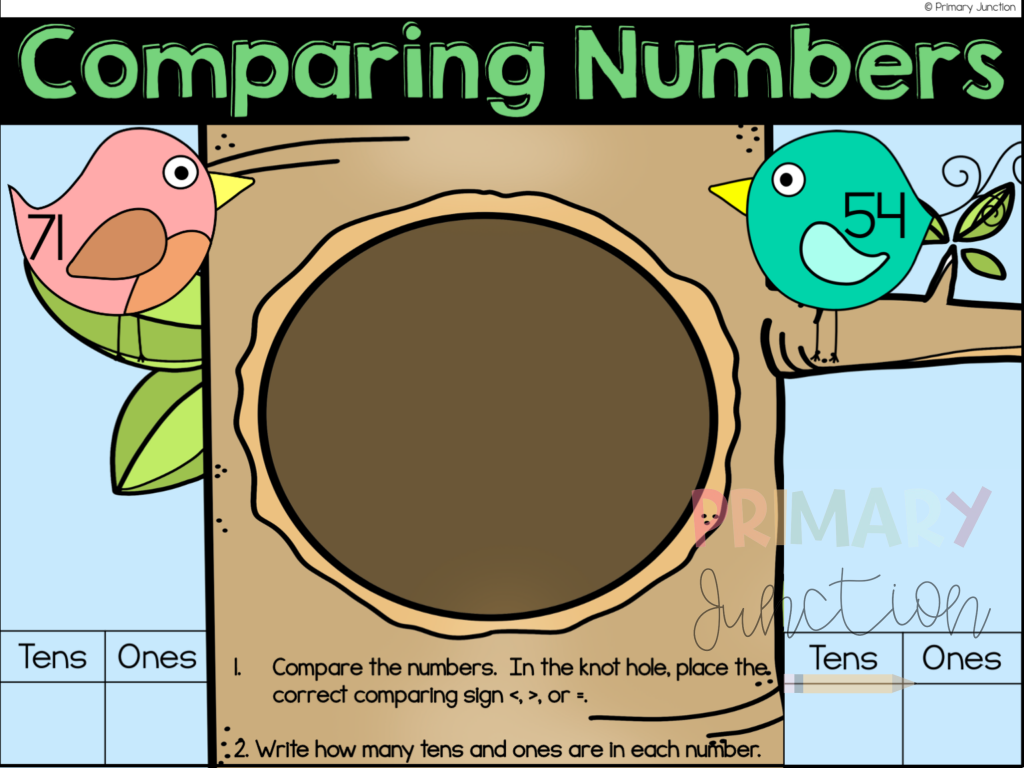3. Buckets of Tens – This activity gives students the opportunity to practice identifying tens in a number. Students are shown a two-digit number and must write the number of tens in the given number.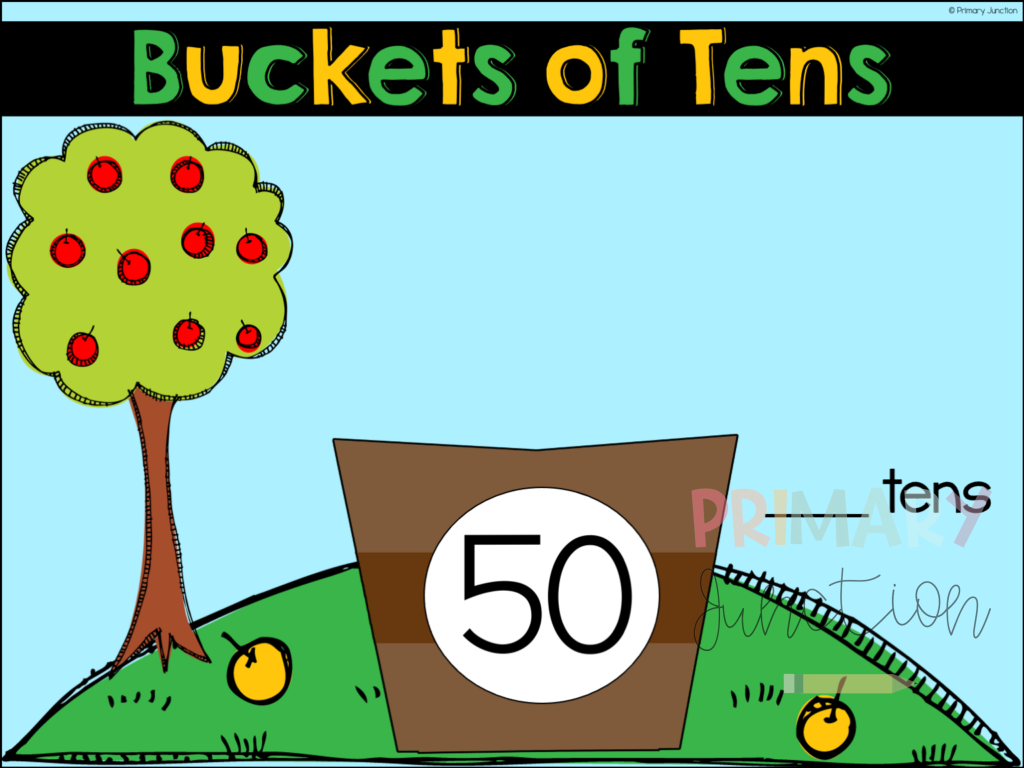4. Build the Number – In this activity, students are shown a two-digit number. They will then build the number using base ten blocks. After building the number, students will identify and write how many tens and ones compose the given number.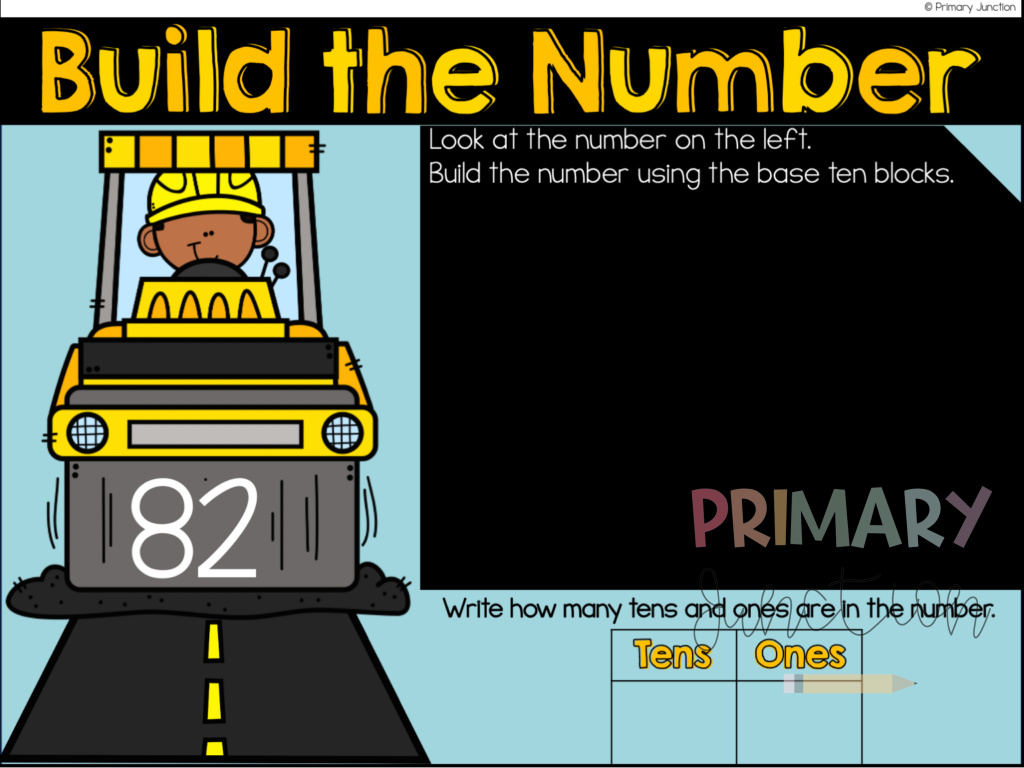5. Frying Up Tens – This activity provides students with the opportunity to practice identifying tens when shown base ten blocks. Students will need to write the value/ones of the given number.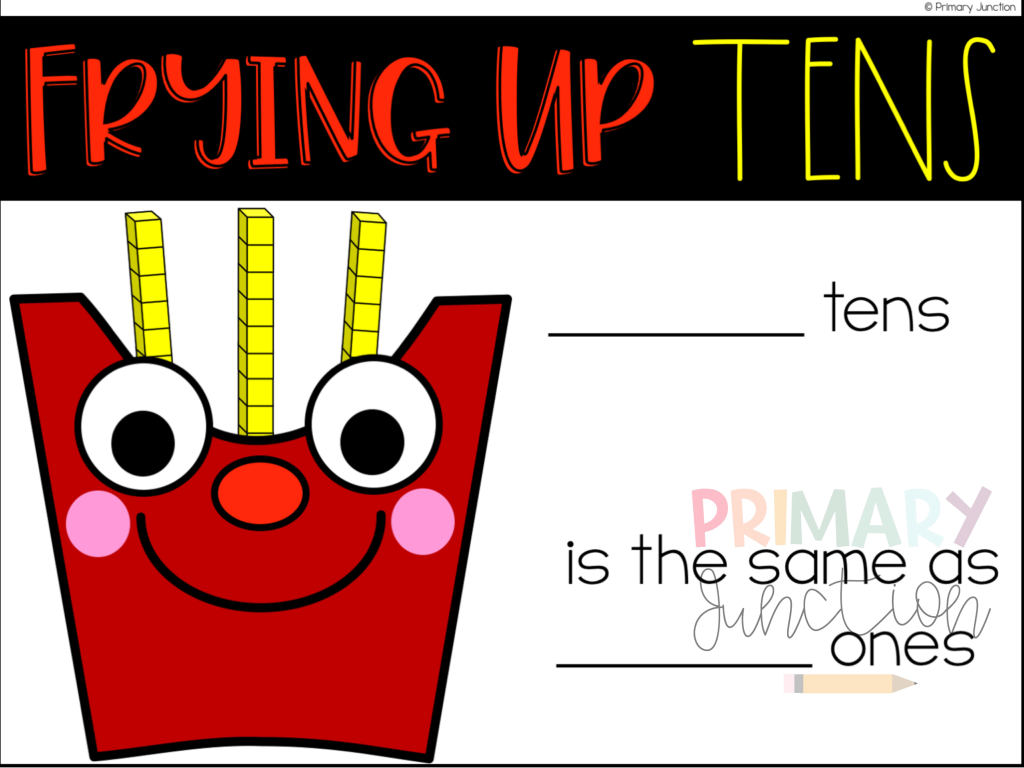6. Groups of Ten – In this activity, students are shown groups of ten using ten frames. Students will write how many groups of ten are shown, as well as the value/ones of the number shown.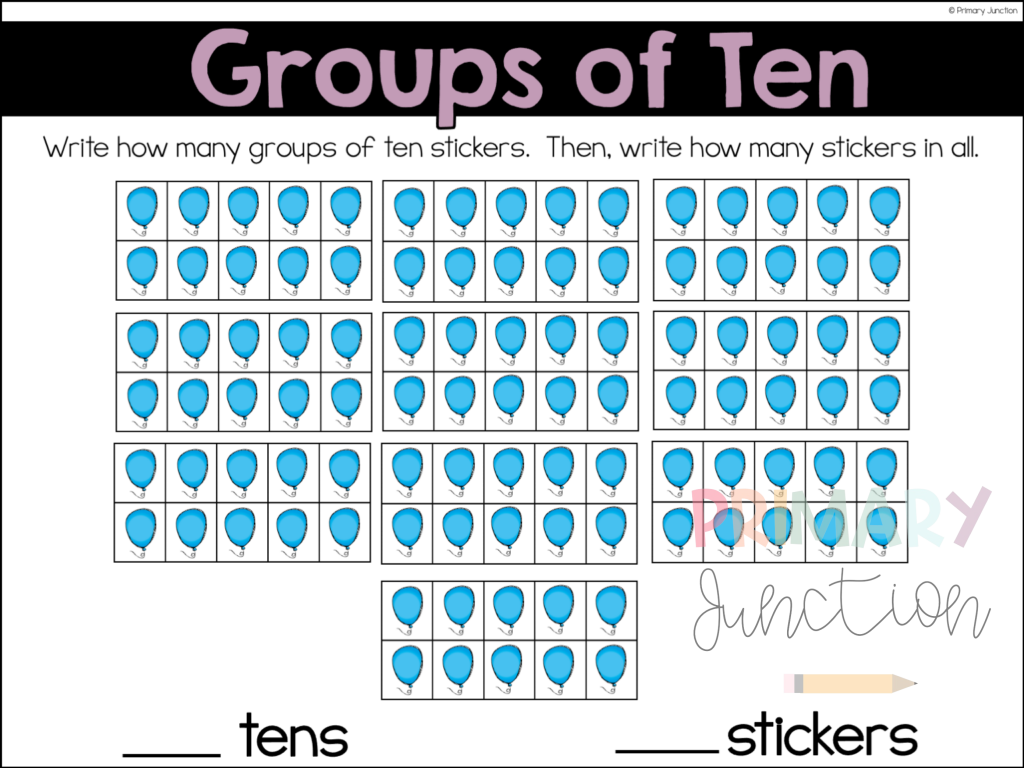7. What is the Number? – This place value tens and ones activity provides students with the opportunity to practice identifying a number when shown base ten blocks/tens and ones.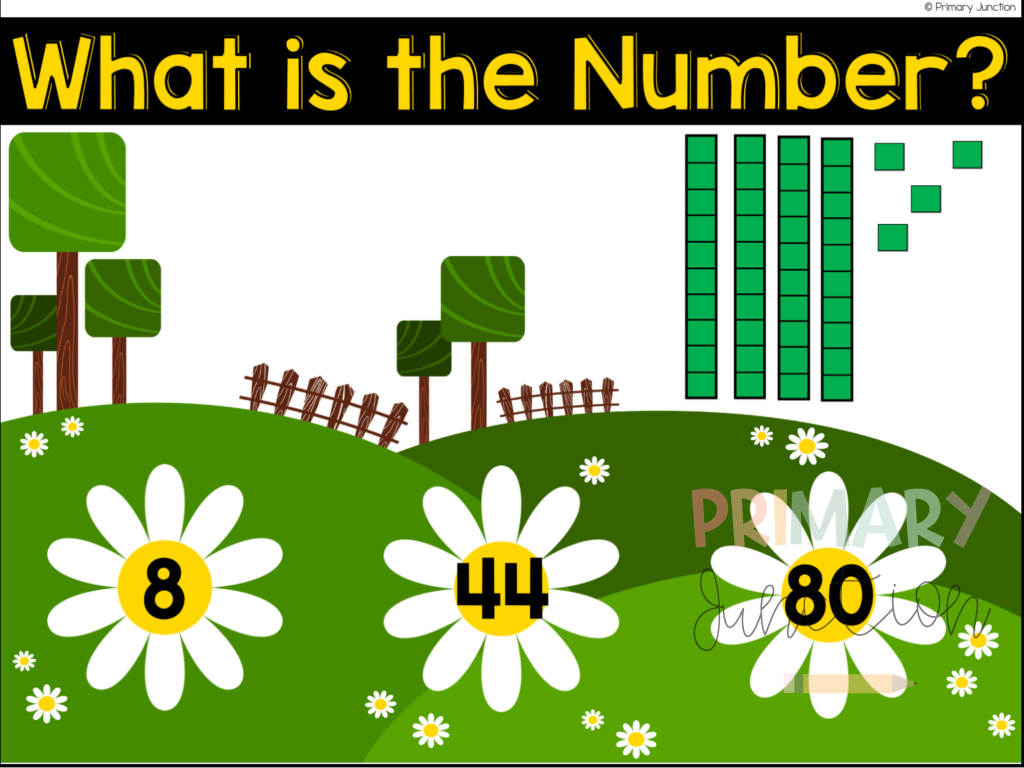8. What is the Number? Tens and Ones – In this activity, students are shown base ten blocks. Students must identify how many tens and ones there are and write the value.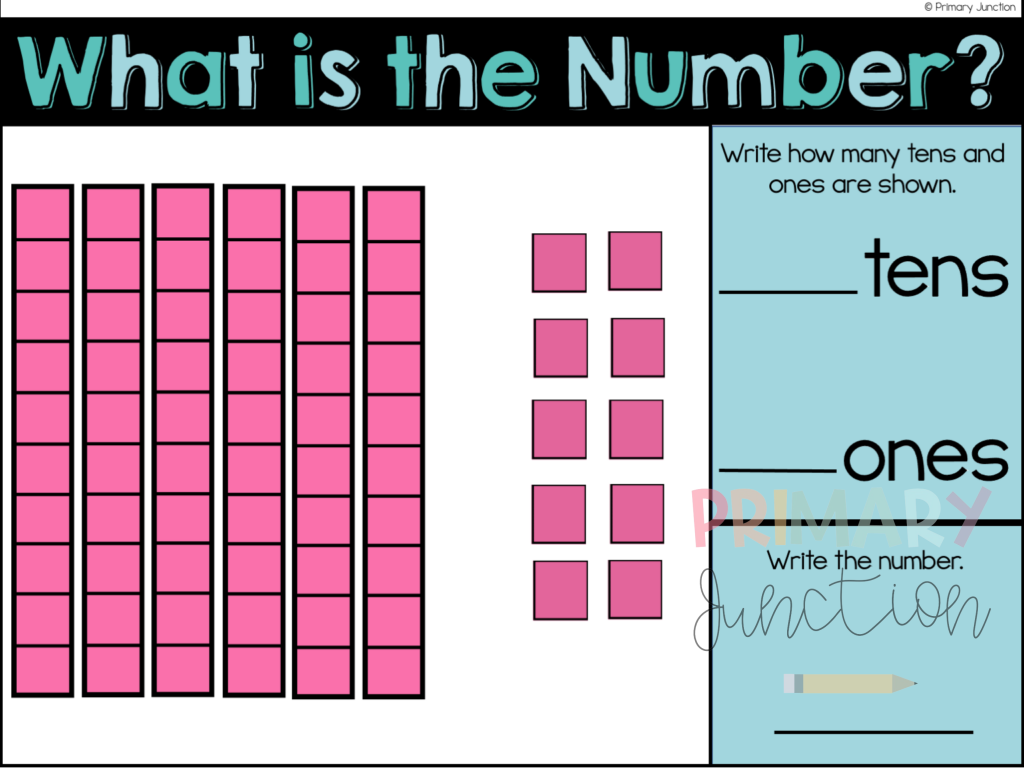### How Do I Use These Place Value Tens and Ones Digital Activities in My Classroom?

Digital math activities are very easy to use!  You can incorporate these activities into whole group instruction, morning work, exit tickets, early finishers, math skill practice, math centers, or even homework practice! These no-prep digital math activities are ready to use in PowerPoint or assign in Google Slides™️ or Seesaw™️, and video directions are also included on how to access the files and assign them to your students.  Easy, step-by-step written student directions are also included with each activity.

SeeSaw: The eight activities are preloaded into Seesaw. You will simply need to click to save the activities to your Seesaw library and assign them to your students.

PowerPoint: The eight files are included in this purchase. You can simply open the files to display to your students or share them through screen share.

#### What Device Do My Students Need?

These place value tens and ones digital math activities will work on any device such as tablets, Smartboards, desktop computers, laptops, Chromebooks, and even smartphones! You will just need to have access to PowerPoint, Google Slides, or SeeSaw. Free apps are available for each of those programs.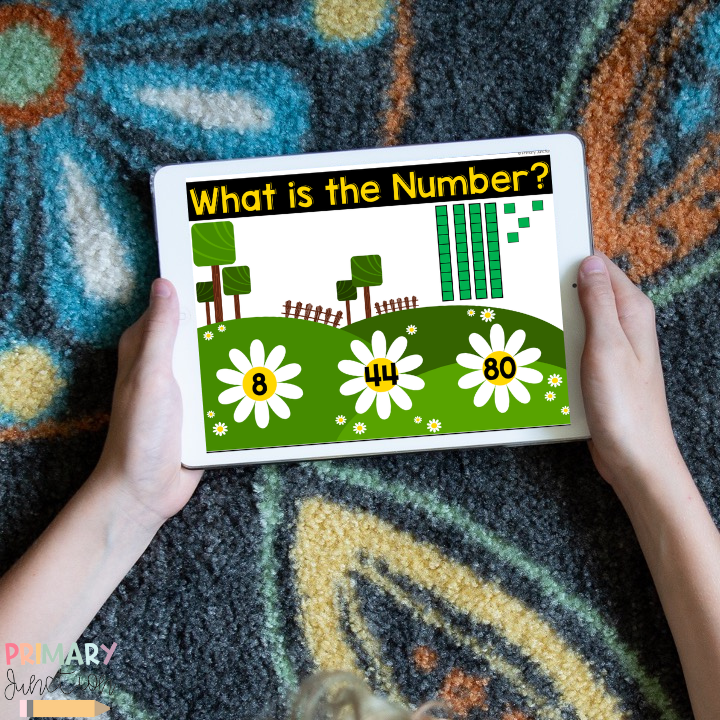Check them out here!

If you have gone digital and are looking for more resources, here are a few more digital ideas:

Digital Math Activities for Composing and Decomposing Teen Number